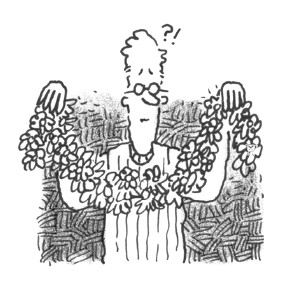### Home > CALC > Chapter 10 > Lesson 10.1.4 > Problem10-36

10-36.

Jules wants to hang a garland in the shape of $f(x) = 2(x^2 + 1)^{−2}$ for $−2 ≤ x ≤ 2$ when $x$ is measured in feet. How long does his garland need to be?The general formula for arc length is:
Use a calculator to complete the integration.